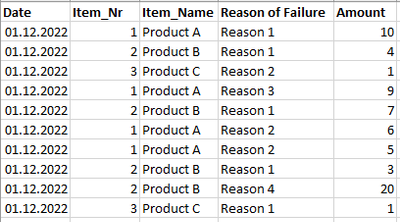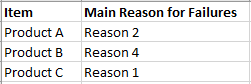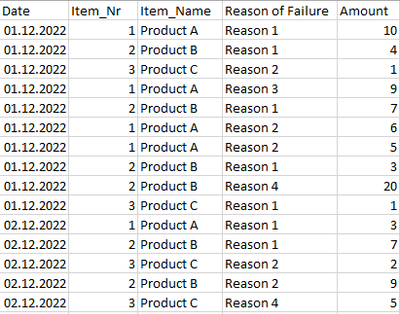cancel
Showing results for
Did you mean:Regular Visitor

## Calculating the top value per summed up category

Hello,

I'm looking for a Measure, that returns me the main reason why a certain Item fails in a filtered timespan.

The measure should return a single string, so it can be used in a matrix visual.

I created Sample Data to reconstruct the problem:The relations look like this:The expected output of the measure "Main Reason for Failure" should return the following given the filter is set to the 01.12.2022:My last attempt at this was with MAXX and TOPN. The problem with this is, that it would return Reason 1 for Product A as Main Failure, since it is the largest single amount booked.

But i would need the measure to sum up all bookings in the filtered time span and then return the main failure per product according to the highest sum of amounts over all bookings (6 + 5 > 10 --> Reason 2 for Product A)

If there two reasons have the same sum, it can show either one (like Product C). Its not critical which it shows, it should just not crash the table 🙂

Any help or recommandations appreciated!

Best Regards

Daniel Schultz

2 REPLIES 2Community Support

You can try this method:

Create a summarized table first:

``````Table 2 =
SUMMARIZE (
SELECTCOLUMNS (
'Table',
"Item_Name", 'Table'[Item_Name],
"RF", 'Table'[Reason of Failure],
"A",
CALCULATE (
SUM ( 'Table'[Amount] ),
FILTER (
'Table',
'Table'[Item_Name] = EARLIER ( 'Table'[Item_Name] )
&& 'Table'[Reason of Failure] = EARLIER ( 'Table'[Reason of Failure] )
)
)
),
[A],
[Item_Name],
[RF]
)
``````

Then new a measure in the first table:

``````Main Reason for Failures =
VAR _max =
CALCULATE (
MAX ( 'Table 2'[A] ),
ALLEXCEPT ( 'Table 2', 'Table 2'[Item_Name] )
)
RETURN
CALCULATE ( MAX ( 'Table 2'[RF] ), FILTER ( 'Table 2', _max = [A] ) )
``````

The result is:Hope this helps you.

Here is my PBIX file.

Best Regards,

Community Support Team _Yinliw

If this post helps, then please consider Accept it as the solution to help the other members find it more quickly.Regular Visitor

Thank you already for the idea with the extra table - it helped.

Sadly it didnt solve the problem completely, since the Main Reason for Failures should also consider active filters on the time and only show the Main Reason for Failures in the filtered time.

To explain this better i added some lines (i should have done this beforehand alraedy - sorry for that).

This is the current input data:Relations stay the same of course and exactly like you did it should be shown in a matrix 🙂

These are the expected results with the respective active filters on the date-collumn. Probably its just some addition to your measure that i can't figure out...